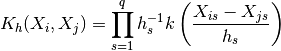# statsmodels.nonparametric.kernel_density.KDEMultivariate.pdf¶

KDEMultivariate.pdf(data_predict=None)[source]

Evaluate the probability density function.

Parameters: data_predict: array_like, optional Points to evaluate at. If unspecified, the training data is used. pdf_est: array_like Probability density function evaluated at data_predict.

Notes

The probability density is given by the generalized product kernel estimator: